# 13.1. Group creation routines

zeros(shape, dtype=<type 'float'>)

Return a new group of given shape and type, filled with zeros.

Parameters: shape (tuple) – Shape of the new group, e.g., (2, 3) or 2. dtype – The desired data-type for the group, e.g.,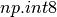. Default is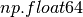. Group with the given shape and dtype filled with zeros.

Examples

```>>> dana.zeros((2,2))
Group([[0.0, 0.0],
[0.0, 0.0]],
dtype=[('f0', '<f8')])
>>> dana.zeros((2,2), dtype=int)
Group([[0, 0],
[0, 0]],
dtype=[('f0', '<f8')])
```

ones(shape, dtype=<type 'float'>)

Return a new group of given shape and type, filled with ones.

Parameters: shape (tuple) – Shape of the new group, e.g., (2, 3) or 2. dtype – The desired data-type for the group, e.g.,. Default is. Group with the given shape and dtype filled with zeros.

Examples

```>>> dana.ones((2,2))
Group([[1.0, 1.0],
[1.0, 1.0]],
dtype=[('f0', '<f8')])
>>> dana.ones((2,2), dtype=int)
Group([[1, 1],
[1, 1]],
dtype=[('f0', '<f8')])
```

empty(shape, dtype=<type 'float'>)

Return a new group of given shape and type, without initialising entries.

Parameters: shape (tuple) – Shape of the new group, e.g., (2, 3) or 2. dtype – The desired data-type for the group, e.g.,. Default is. Group with the given shape and dtype filled with zeros.

Notes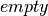, unlike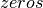, does not set the group values to zero, and may therefore be marginally faster. On the other hand, it requires the user to manually set all the values in the group, and should be used with caution.

Examples

```>>> Group.empty((2,2))
Group([[6.94248367807e-310, 1.34841898023e-316],
[1.34841977073e-316, 0.0]],
dtype=[('f0', '<f8')])
```

zeros_like(other)

Create a new group of zeros with the same shape and type as another.

Parameters: other (array) – The shape and data-type of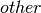defines the parameters of the returned group. Group of zeros with same shape and type as.

Examples

```>>> x = np.arange(6)
>>> x = x.reshape((2, 3))
>>> x
array([[0, 1, 2],
[3, 4, 5]])
>>> np.zeros_like(x)
array([[0, 0, 0],
[0, 0, 0]])
```

ones_like(other)

Returns a group of ones with the same shape and type as a given array.

Parameters: other (array) – The shape and data-type ofdefines the parameters of the returned group. Group of ones with same shape and type as.

Examples

```>>> x = np.arange(6)
>>> x = x.reshape((2, 3))
>>> x
array([[0, 1, 2],
[3, 4, 5]])
>>> zeros_like(x)
group([[0, 0, 0],
[0, 0, 0]])
```

empty_like(other)

Create a new group with the same shape and type as another.

Parameters: other (array) – The shape and data-type ofdefines the parameters of the returned group. Uninitialized group with same shape and type as.

Examples

```>>> x = np.arange(6)
>>> x = x.reshape((2, 3))
>>> x
array([[0, 1, 2],
[3, 4, 5]])
>>> np.zeros_like(x)
array([[0, 0, 0],
[0, 0, 0]])
```